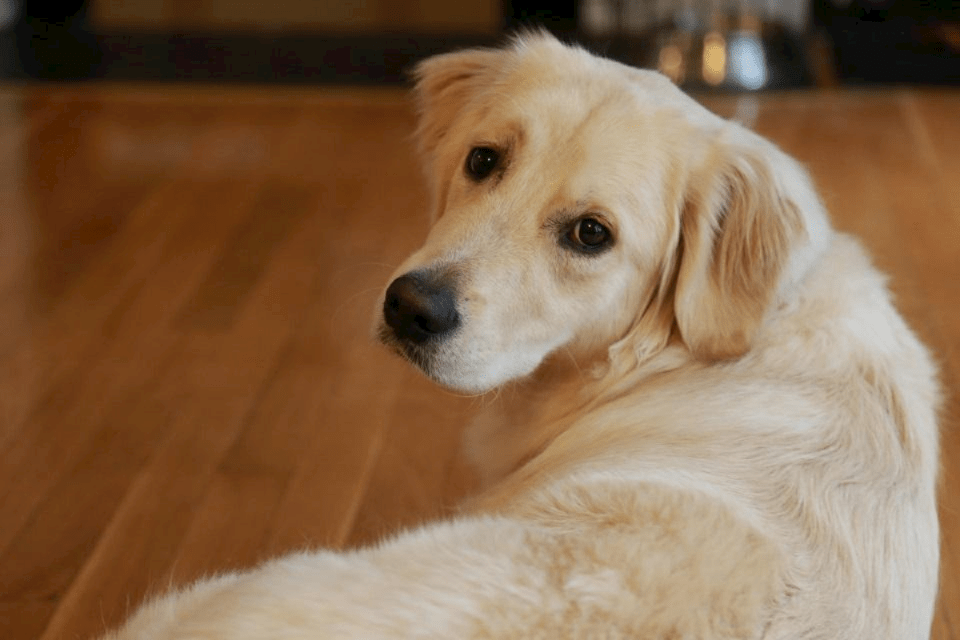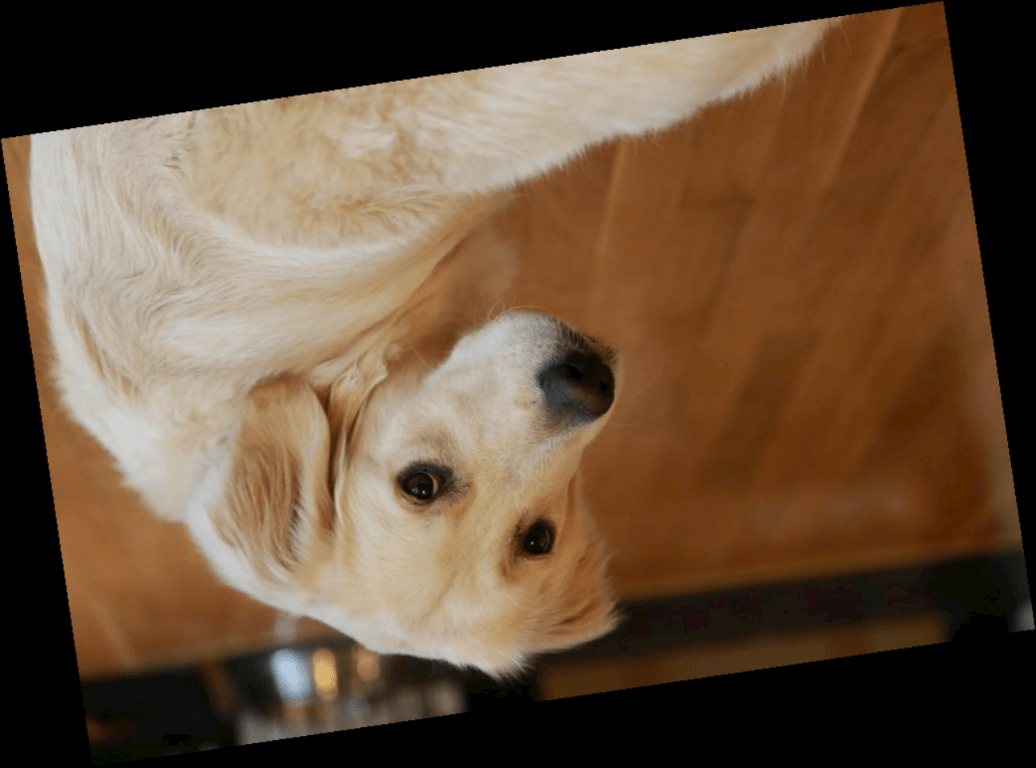# randomAffine2d

Create randomized 2-D affine transformation

Since R2019b

## Syntax

``tform = randomAffine2d``
``tform = randomAffine2d(Name,Value)``

## Description

````tform = randomAffine2d` creates an `affinetform2d` object that performs an identity transformation.```

example

````tform = randomAffine2d(Name,Value)` specifies the type of affine transformation using name-value arguments.```

## Examples

collapse all

```I = imread("kobi.png"); imshow(I)```Create a 2-D affine transformation object that rotates images. The `randomAffine2d` function picks the rotation angle randomly from a continuous uniform distribution within the interval [35, 55] degrees.

`tform1 = randomAffine2d(Rotation=[35 55]);`

Rotate the image and display the result.

```J = imwarp(I,tform1); imshow(J)```The transformation object, `tform1`, rotates all images by the same amount. To rotate an image by a different randomly selected amount, create a new geometric transformation.

```tform2 = randomAffine2d(Rotation=[-10 10]); J2 = imwarp(I,tform2); imshow(J2)``````I = imread("sherlock.jpg"); imshow(I)```Create a 2-D affine transformation object that rotates images. To select a rotation angle from a custom range, specify the `Rotation` name-value argument as a function handle. This example specifies a function called `myrange` (defined at the end of the example) that selects an angle from within two disjoint intervals.

`tform = randomAffine2d(Rotation=@myrange);`

Rotate the image and display the result.

```J = imwarp(I,tform); imshow(J)```Supporting Function

This example defines the `myrange` function that first randomly selects one of two intervals (-10, 10) and (170, 190) with equal probability. Within the selected interval, the function returns a single random number from a uniform distribution.

```function angle = myrange() if randi([0 1],1) a = -10; b = 10; else a = 170; b = 190; end angle = a + (b-a).*rand(1); end```

## Input Arguments

collapse all

### Name-Value Arguments

Specify optional pairs of arguments as `Name1=Value1,...,NameN=ValueN`, where `Name` is the argument name and `Value` is the corresponding value. Name-value arguments must appear after other arguments, but the order of the pairs does not matter.

Example: `tform = randomAffine2d(XReflection=true)`

Before R2021a, use commas to separate each name and value, and enclose `Name` in quotes.

Example: `tform = randomAffine2d("XReflection",true)`

Random horizontal reflection, specified as `false` or `true`. When `XReflection` is `true` (`1`), the transformation `tform` reflects images horizontally with 50% probability. By default, the transformation does not reflect images in the horizontal direction.

Random vertical reflection, specified as `false` or `true`. When `YReflection` is `true` (`1`), the transformation `tform` reflects images vertically with 50% probability. By default, the transformation does not reflect images in the vertical direction.

Range of rotation, in degrees, applied to the input image, specified as one of the following.

• A 2-element numeric vector. The second element must be larger than or equal to the first element. The rotation angle is picked randomly from a continuous uniform distribution within the specified interval.

• A function handle. The function must accept no input arguments and return the rotation angle as a numeric scalar. Use a function handle to pick rotation angles from a disjoint interval or using a nonuniform probability distribution. For more information about function handles, see Create Function Handle.

By default, the transformation `tform` does not rotate images.

Example: `[-45 45]`

Range of uniform (isotropic) scaling applied to the input image, specified as one of the following.

• A 2-element numeric vector. The second element must be larger than or equal to the first element. The scale factor is picked randomly from a continuous uniform distribution within the specified interval.

• A function handle. The function must accept no input arguments and return the scale factor as a numeric scalar. Use a function handle to pick scale factors from a disjoint interval or using a nonuniform probability distribution. For more information about function handles, see Create Function Handle.

By default, the transformation `tform` does not scale images.

Example: `[0.5 4]`

Range of horizontal shear applied to the input image, specified as one of the following. Shear is measured as an angle in degrees, and is in the range (–90, 90).

• A 2-element numeric vector. The second element must be larger than or equal to the first element. The horizontal shear angle is picked randomly from a continuous uniform distribution within the specified interval.

• A function handle. The function must accept no input arguments and return the horizontal shear angle as a numeric scalar. Use a function handle to pick horizontal shear angles from a disjoint interval or using a nonuniform probability distribution. For more information about function handles, see Create Function Handle.

By default, the transformation `tform` does not shear images in the horizontal direction.

Example: `[0 45]`

Range of vertical shear applied to the input image, specified as one of the following. Shear is measured as an angle in degrees, and is in the range (–90, 90).

• A 2-element numeric vector. The second element must be larger than or equal to the first element. The vertical shear angle is picked randomly from a continuous uniform distribution within the specified interval.

• A function handle. The function must accept no input arguments and return the vertical shear angle as a numeric scalar. Use a function handle to pick vertical shear angles from a disjoint interval or using a nonuniform probability distribution. For more information about function handles, see Create Function Handle.

By default, the transformation `tform` does not shear images in the vertical direction.

Example: `[0 45]`

Range of horizontal translation applied to the input image, specified as one of the following. Translation distance is measured in pixels.

• A 2-element numeric vector. The second element must be larger than or equal to the first element. The horizontal translation distance is picked randomly from a continuous uniform distribution within the specified interval.

• A function handle. The function must accept no input arguments and return the horizontal translation distance as a numeric scalar. Use a function handle to pick horizontal translation distances from a disjoint interval or using a nonuniform probability distribution. For more information about function handles, see Create Function Handle.

By default, the transformation `tform` does not translate images in the horizontal direction.

Example: `[-5 5]`

Range of vertical translation applied to the input image, specified as one of the following. Translation distance is measured in pixels.

• A 2-element numeric vector. The second element must be larger than or equal to the first element. The vertical translation distance is picked randomly from a continuous uniform distribution within the specified interval.

• A function handle. The function must accept no input arguments and return the vertical translation distance as a numeric scalar. Use a function handle to pick vertical translation distances from a disjoint interval or using a nonuniform probability distribution. For more information about function handles, see Create Function Handle.

By default, the transformation `tform` does not translate images in the vertical direction.

Example: `[-5 5]`

## Output Arguments

collapse all

Affine transformation, returned as an `affinetform2d` object.

## Version History

Introduced in R2019b

expand all